Latest Banking jobs   »   Reasoning Ability Quiz For SBI Clerk...

# Reasoning Ability Quiz For SBI Clerk Prelims 2021- 13th May

Q1. A person starts walking towards point Y which in North direction of starting point. From point Y, he start walking in west direction then he takes right turn to reach at point W. From point W, he takes a right turn and stop at point J. Find in which direction person is facing now?
(a) North
(b) South
(c) East
(d) West
(e) None of these

Q2. Point H is 5m west of J. Point J is 10m south of point T. Point T is 12m west of G. Point G is 6m north of Point D. Point D is 15m east of Point Y. What is the shortest distance between Point J and Point Y?
(a) 10m
(b) 4m
(c) 9m
(d) 7m
(e) None of these

Directions (3-7): Study the information carefully and answer the questions given below.
Amit starts his journey from point S, walks 20m towards west to reach at point D, then takes a left turn and walks for 8m to reach point N. From point N he takes a left turn and walks for 18m to reach at point X. From point X, he takes a left turn and walks 12m to reach at point Y, then takes a right turn and walks 6m to reach point T. From point T, He walks 4m in south direction and reached point M.

Q3. What is the shortest distance between point S and M?
(a) 4m
(b) 6m
(c) 2m
(d) 5m
(e) None of these

Q4. If point H is 6m west of point M then in which direction point Y with respect to point H?
(a) South
(b) North-west
(c) South-east
(d) East
(e) North

Q5. In which direction point T with respect of D?
(a) North
(b) South-west
(c) North-east
(d) East
(e) South-east

Q6. What is the shortest distance between Point D and Point X?
(a) √388m
(b) 10m
(c) 15m
(d) 20m
(e) None of these

Q7. In which direction point N is with respect of Y?
(a) North-east
(b) South-west
(c) North-west
(d) south
(e) None of these

Directions (8-10): Study the information carefully and answer the questions given below.
Point A is 12m west of point B. Point F is 8m east of point D. Point G is 10m north of point E. Point C is 8m south of point B. Point D is 3m north of point C. Point E is 6m west of point C.

Q8. What is the shortest distance between D and A?
(a) 13m
(b) 10m
(c) 12m
(d) 14m
(e) None of these

Q9. Point D is in which direction with respect to G?
(a) North-East
(b) South-west
(c) East
(d) North
(e) South-east

Q10. If Point Y is 7m south of point G than what is the shortest distance between Point Y and Point F?
(a) 16m
(b) 14m
(c) 13m
(d) 10m
(e) None of these

Directions (11-13): Study the information carefully and answer the questions given below.
Dheeraj starts his journey from point S, walks 10m towards North to reach at point D, then takes a right turn and walks 6m to reach point F. From point F, He start walking in west direction and walk 4m to reach at point N, From point N he takes a left turn and walks 9m to reach at point M, then takes a left turn again and walks 4m to reach point T.

Q11. What is the shortest distance between point T and F?
(a) 6m
(b) 5m
(c) 8m
(d) 9m
(e) None of these

Q12. In which direction point N with respect to T?
(a) North
(b) North east
(c) South east
(d) North west
(e) Can’t be determined

Q13. If point K is 2m north of point S and the shortest distance between point K and F is 10m then, what is the distance between point D and K?
(a) 6m
(b) 8m
(c) 3m
(d) 10m
(e) None of these

Directions (14-15): Study the information carefully and answer the questions given below.
A person starts walking from point X to the east direction, after walking of 5km to reach point P from there he turns towards left and then walk 4km to reach point Q. Then turn to his right and walk 5km to reach point R then turn to his right and walk 4km to reach point S then move towards his left and walk 3km to reach point T then again turn right and walk 3km to reach point U finally turn to his right and walk 8km to reach point Y.

Q14. How far (Shortest Distance) and which direction is point X with respect to point Y?
(a) √30 km North
(b) 34 km North-East
(c) 2√34 km South-West
(d) √34 km North-West
(e) None of these.

Q15. In which direction is point T with respect to point Y?
(a) North east
(b) South east
(c) North
(d) West
(e) South west

Practice More Questions of Reasoning for Competitive Exams:

###### SBI Clerk Study Plan 2021

Solutions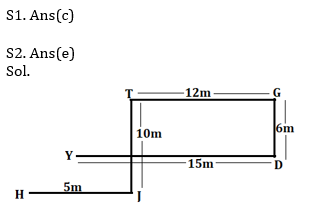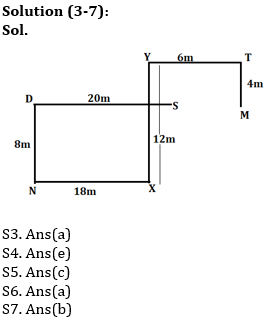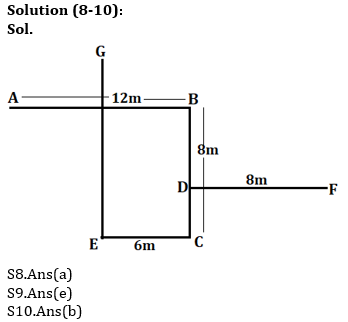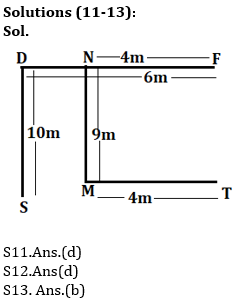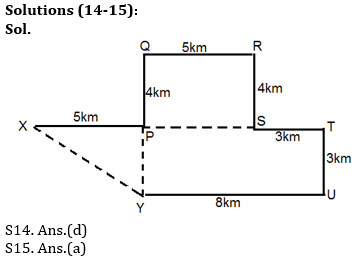#### Congratulations!Incorrect details? Fill the form again here

•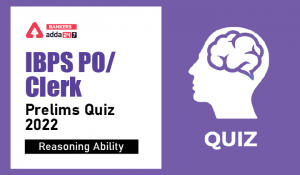Reasoning Ability Quiz For IBPS Clerk/PO...
•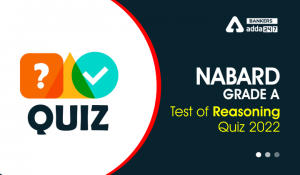Reasoning Ability Quiz For NABARD Grade ...
•Reasoning Ability Quiz For NABARD Grade ...
•Reasoning Ability Quiz For IBPS RRB PO C...
•Reasoning Ability Quiz For IBPS Clerk Pr...
•Reasoning Ability Quiz For IBPS RRB PO P...